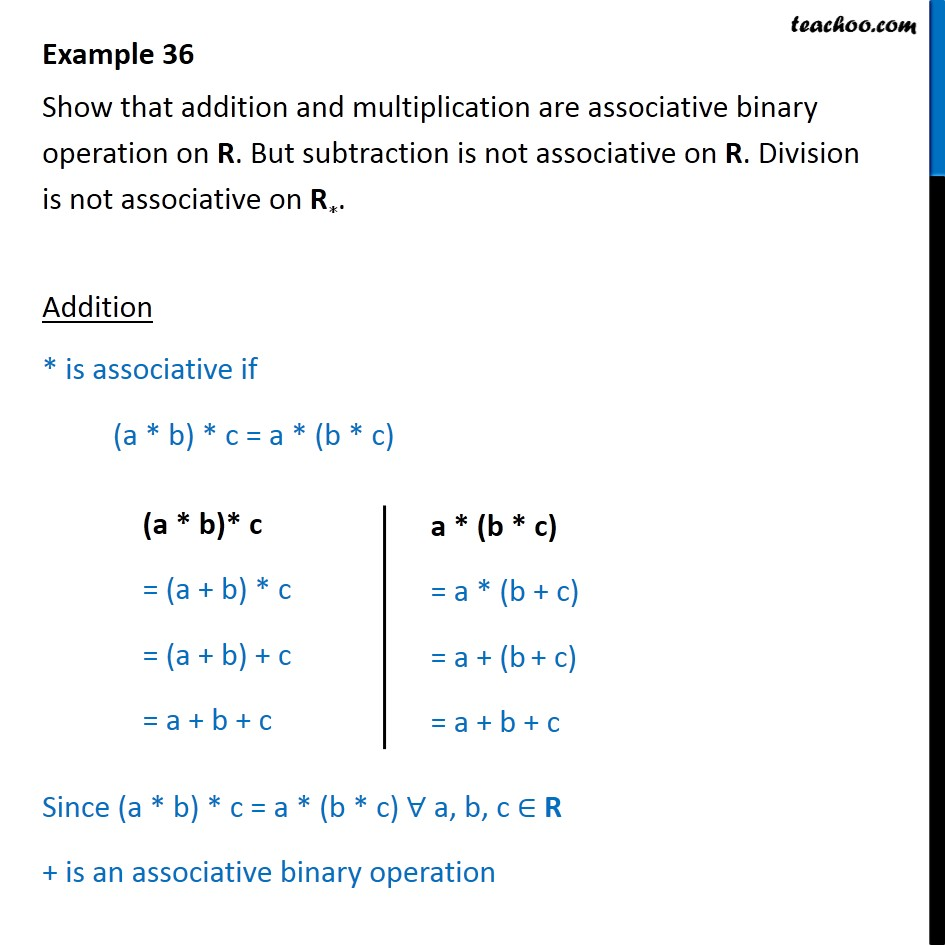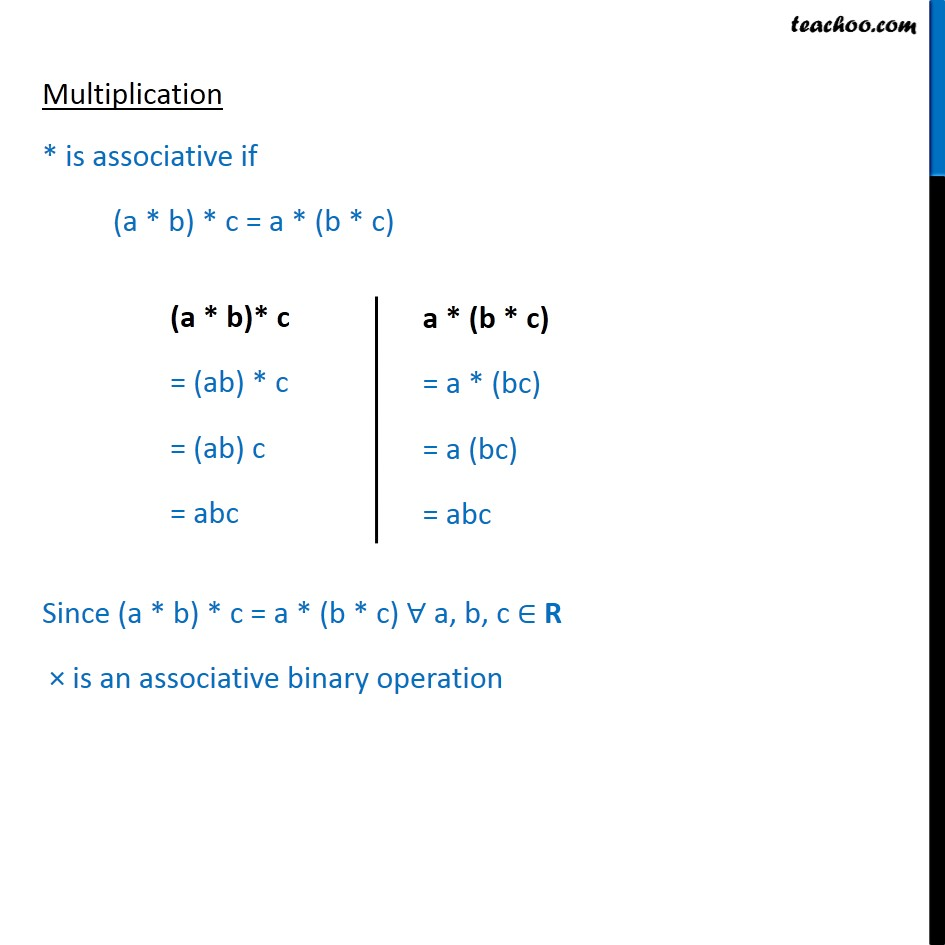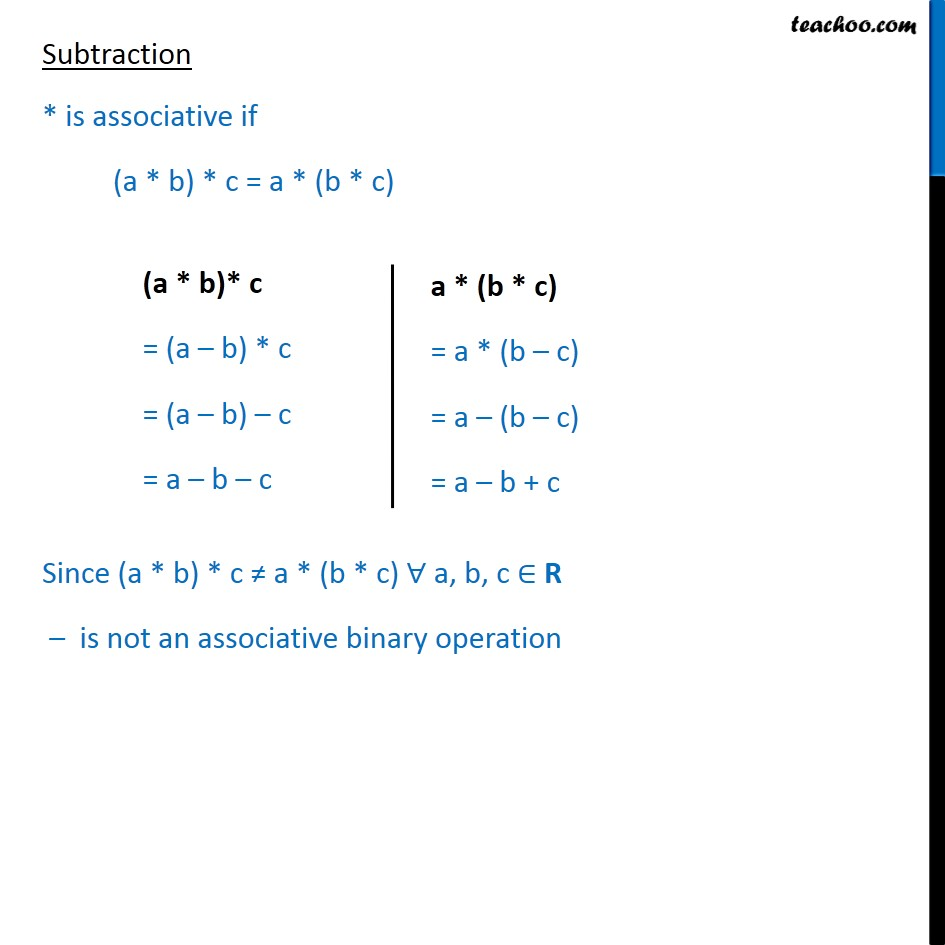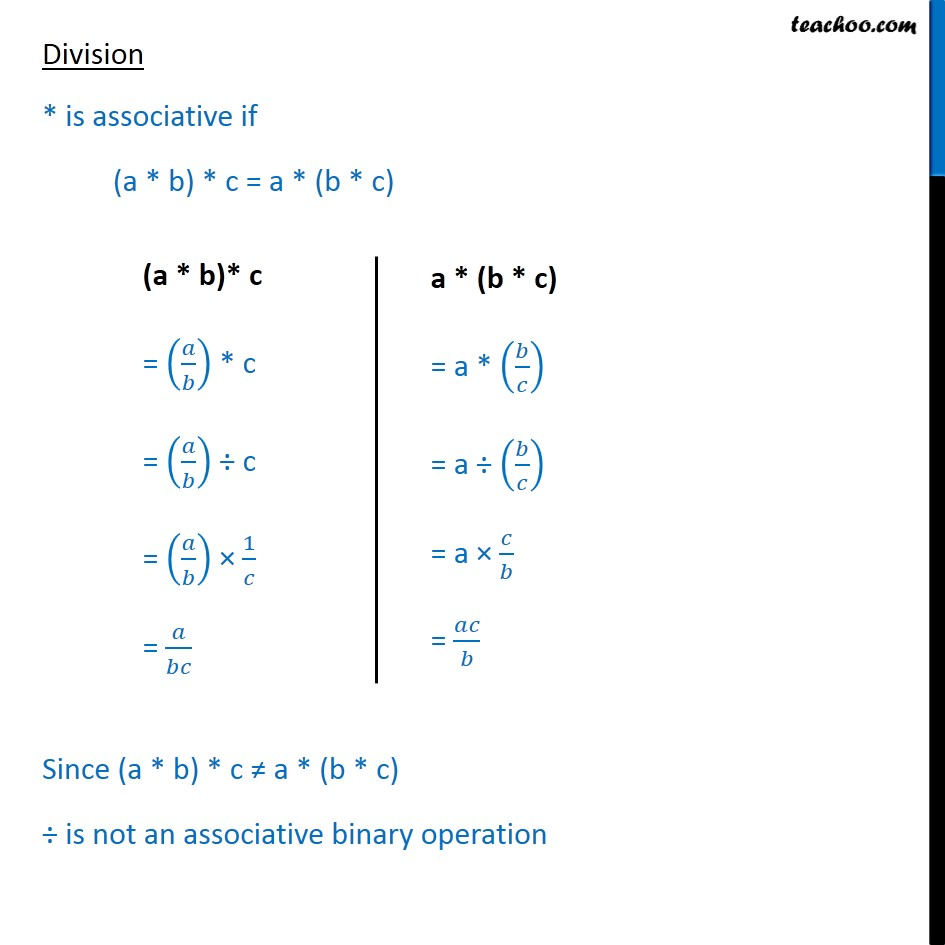Examples

Chapter 1 Class 12 Relation and Functions
Serial order wiseLearn in your speed, with individual attention - Teachoo Maths 1-on-1 Class

### Transcript

Question 19 Show that addition and multiplication are associative binary operation on R. But subtraction is not associative on R. Division is not associative on R∗. Addition * is associative if (a * b) * c = a * (b * c) Since (a * b) * c = a * (b * c) ∀ a, b, c ∈ R + is an associative binary operation Multiplication * is associative if (a * b) * c = a * (b * c) Since (a * b) * c = a * (b * c) ∀ a, b, c ∈ R × is an associative binary operation Subtraction * is associative if (a * b) * c = a * (b * c) Since (a * b) * c ≠ a * (b * c) ∀ a, b, c ∈ R – is not an associative binary operation Division * is associative if (a * b) * c = a * (b * c) Since (a * b) * c ≠ a * (b * c) ÷ is not an associative binary operation